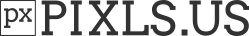# counting pixels / loops

A bit of basic g’mic script syntax question I guess.

But I try to count pixels matching a certain condition.

So I first set a variable to 0, and then with eval wanted to run an expression that increments that variable.

But the variable cannot be accessed ‘live’ it seems. It’s substituted before the eval commands run, right? So as far as eval sees, it’s a constant value, not a variable that can be increments.

How can I do this efficiently?
Otherwise, I’m thinking of something like a for (x=0;x<w;x++) loop inside a for (y=0;y<w;y++), and no eval at all, which doesn’t seem the best approach, or is it?

What I’m trying to do (because maybe there is another way), is to get the average (or the median maybe) value of pixels, but from a masked image. (So I have a single grey channel, and I have a mask with 0 or 255 values, and I only want the average of pixels where the mask is 255).

My suggestion is to proceed in two steps:

1. Extract the pixel values you are interested in, as a new image.
2. Compute the average/median colors of this new image.

Step 1 can be done using the comand extract.
Assuming your image  is your color image and  is a binary mask, you can do something as:

+append[0,1] c extract[-1] "A!=0",3


This inserts a new image () with size 1\times N\times 1\times 3 containing only the color pixels where the mask value is not zero.

Then:

avg_color=${-average_vectors[-1]} med_color=${-median_color[-1]}


returns the average and median color (computed channel by channel).

but if I’m reading this right,

‘extract’ takes pixels based on an evaluation, and dumps all those pixels together in an image with 1px wide and then N pixels tall. (weird, why not N wide and 1 tall as in other 1-dimensional images?).

In your example the R, G and B values are then put ‘in depth’ (which I hardly touched on in g’mic to be honest). For now, I’m working on greyscale images (the A and the B channels of an OkLab image separately), but I can use the same approach I guess.

Intriguing, this can indeed make it way simpler and hopefully faster than what I have now (a for loop that just goes over the offsets of the source image).

Hmm… Since I have one greyscale (single channel, #0) image with values, and another one with the binary mask (#1)… I was hoping that I could do something like +extract "i#1>0",2 to get a simple 1xNx1x1 image with all the values where the mask is not black.

But the i#1 doesn’t seem to work like that there :(.
If I append the two images together (+append[0,1] c) and then run extract on that (extract. "i1>0",2) it also does something different than I hoped. The resulting image is now 1x(N*2)x1x1, because I first get all the values from the original image (1st channel) and then all the selected values from the mask (2nd channel).

Using vectors like you did, does work (I get a 1xNx1x2 image with the values I expect). But I now have to somehow lose the 2nd values from the vectors / 2nd layer.

No, the expression in extract is somehow limited to using the current image features, that’s why I’ve appended the image and the mask at first before calling extract in my example.

If you want to use features from another images, here are another (more flexible and generic) solutions:

foo :
# Solution 1 : Extract pixels of interest, using dynamic arrays,
# then compute average and median colors.
1,1,1,3 # Dynamic array to store RGB values
eval "i?da_push(I(#0));
end(resize(#-1,1,da_size(),1,s#-1,0))"
avg_color=${-average_vectors.} med_color=${-median_color.}
rm.
e $avg_color # Solution 2 : Direct computation of the average without pixel extraction. # (cannot be used for median color though). eval ">begin(sum = vector(#s#0); N = 0); i?(sum+=I(#0); ++N); end(set('avg_color',vtos(sum/N)))" e$avg_color


I had trouble dealing with the vectors extract gives as output.

So i do the same as you , i append my image and the mask together (but it’s a grey image so i now have two channels ).

The eval code with A didn’t work. I guess it’s a simple alias for i3 and that doesn’t work in my 2 channels. So your eval code with the A replaced by it worked fine.

So now I have an output image, where every value is a vector: the image source value, and 255 for the mask value.

How i convert those vectors to just simple scalars of the first value? (‘dumling the 255’).

What i ended up doing was using extract in scalar output mode , and cropping it’s output image to only use the top half. But this feels a bit clunky.

I thought I could somehow split those vectors and only use the first value… Or use a fill to replace the vectors eith a scalar value, but I can’t seem to manage it. I don’t know how to access the vector that is ‘the current pixel’. Skmrthing like fill. "i" does ofcourse something completely different (taking the first pixel, instead of taking the current pixel’ but the first value from the vector )

Indeed, you should use i1 in your case.

channels 0 seems to be the solution here.

Ohhhh, I’m overthinking the whole ‘vectors’ thing, isn’t it.

I see that a regular RGB image is also a ‘w, h, 1, 3’ type image, with R/G/B displayed as a vector [ R, G, B] in the preview.

So my ‘extract’ output image is actually a ‘w, h, 1, 2’ type image (d’uh), with V, A displayed as a vector [ V, A ].

So indeed, I can just drop the 2nd channel to only get the first value.

Yes, vector-valued images are just images with a number of channels N>1, and each pixel is then considered as a vector of size N.